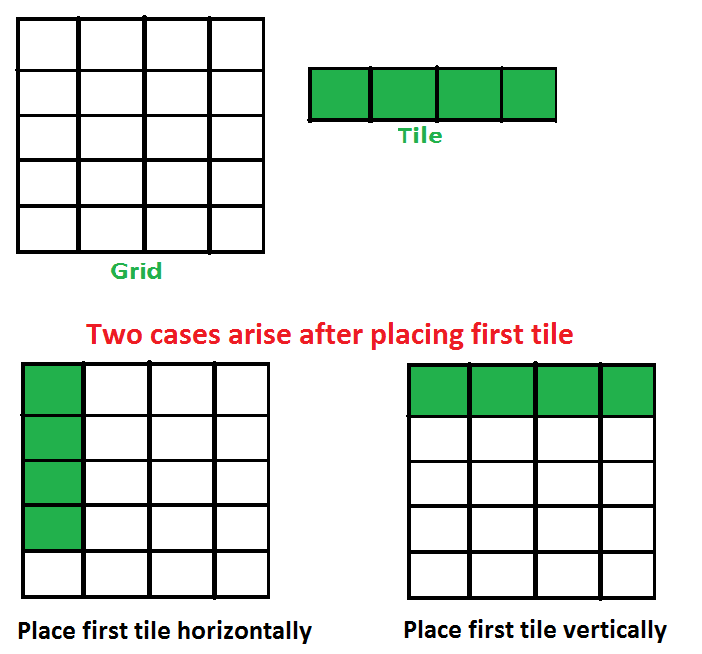Open in App
Not now

# Count number of ways to fill a “n x 4” grid using “1 x 4” tiles

• Difficulty Level : Medium
• Last Updated : 05 Jul, 2022

Given a number n, count number of ways to fill a n x 4 grid using 1 x 4 tiles.
Examples:

```Input : n = 1
Output : 1

Input : n = 2
Output : 1
We can only place both tiles horizontally

Input : n = 3
Output : 1
We can only place all tiles horizontally.

Input : n = 4
Output : 2
The two ways are :
1) Place all tiles horizontally
2) Place all tiles vertically.

Input : n = 5
Output : 3
We can fill a 5 x 4 grid in following ways :
1) Place all 5 tiles horizontally
2) Place first 4 vertically and 1 horizontally.
3) Place first 1 horizontally and 4 vertically.```

## We strongly recommend that you click here and practice it, before moving on to the solution.

This problem is mainly an extension of this tiling problem
Let “count(n)” be the count of ways to place tiles on a “n x 4” grid, following two cases arise when we place the first tile.

1. Place the first tile horizontally : If we place first tile horizontally, the problem reduces to “count(n-1)”
2. Place the first tile vertically : If we place first tile vertically, then we must place 3 more tiles vertically. So the problem reduces to “count(n-4)”Therefore, count(n) can be written as below.

```   count(n) = 1 if n = 1 or n = 2 or n = 3
count(n) = 2 if n = 4
count(n) = count(n-1) + count(n-4) ```

This recurrence is similar to Fibonacci Numbers and can be solved using Dynamic programming.

## C++

 `// C++ program to count of ways to place 1 x 4 tiles``// on n x 4 grid.``#include``using` `namespace` `std;` `// Returns count of count of ways to place 1 x 4 tiles``// on n x 4 grid.``int` `count(``int` `n)``{``    ``// Create a table to store results of subproblems``    ``// dp[i] stores count of ways for i x 4 grid.``    ``int` `dp[n+1];``    ``dp = 0;` `    ``// Fill the table from d to dp[n]``    ``for` `(``int` `i=1; i<=n; i++)``    ``{``        ``// Base cases``        ``if` `(i >= 1 && i <= 3)``            ``dp[i] = 1;``        ``else` `if` `(i==4)``            ``dp[i] = 2 ;` `        ``else``            ``// dp(i-1) : Place first tile horizontally``            ``// dp(n-4) : Place first tile vertically``            ``//         which means 3 more tiles have``            ``//         to be placed vertically.``            ``dp[i] = dp[i-1] + dp[i-4];``    ``}` `    ``return` `dp[n];``}` `// Driver program to test above``int` `main()``{``    ``int` `n = 5;``    ``cout << ``"Count of ways is "` `<< count(n);``    ``return` `0;``}`

## Java

 `// Java program to count of ways to place 1 x 4 tiles``// on n x 4 grid``import` `java.io.*;` `class` `Grid``{``    ``// Function that count the number of ways to place 1 x 4 tiles``    ``// on n x 4 grid.``    ``static` `int` `count(``int` `n)``    ``{``        ``// Create a table to store results of sub-problems``        ``// dp[i] stores count of ways for i x 4 grid.``        ``int``[] dp = ``new` `int``[n+``1``];``        ``dp[``0``] = ``0``;``        ``// Fill the table from d to dp[n]``        ``for``(``int` `i=``1``;i<=n;i++)``        ``{``            ``// Base cases``            ``if` `(i >= ``1` `&& i <= ``3``)``                ``dp[i] = ``1``;``            ``else` `if` `(i==``4``)``                ``dp[i] = ``2` `;` `            ``else``            ``{``                    ``// dp(i-1) : Place first tile horizontally``                    ``// dp(i-4) : Place first tile vertically``                    ``//         which means 3 more tiles have``                    ``//         to be placed vertically.``                    ``dp[i] = dp[i-``1``] + dp[i-``4``];``            ``}``        ``}``        ``return` `dp[n];``    ``}``    ` `    ``// Driver program``    ``public` `static` `void` `main (String[] args)``    ``{``        ``int` `n = ``5``;``        ``System.out.println(``"Count of ways is: "` `+ count(n));``    ``}``}` `// Contributed by Pramod Kumar`

## Python3

 `# Python program to count of ways to place 1 x 4 tiles``# on n x 4 grid.` `# Returns count of count of ways to place 1 x 4 tiles``# on n x 4 grid.``def` `count(n):` `    ``# Create a table to store results of subproblems``    ``# dp[i] stores count of ways for i x 4 grid.``    ``dp ``=` `[``0` `for` `_ ``in` `range``(n``+``1``)]` `    ``# Fill the table from d to dp[n]``    ``for` `i ``in` `range``(``1``,n``+``1``):` `        ``# Base cases``        ``if` `i <``=` `3``:``            ``dp[i] ``=` `1``        ``elif` `i ``=``=` `4``:``            ``dp[i] ``=` `2``        ``else``:``            ``# dp(i-1) : Place first tile horizontally``            ``# dp(n-4) : Place first tile vertically``            ``#           which means 3 more tiles have``            ``#           to be placed vertically.``            ``dp[i] ``=` `dp[i``-``1``] ``+` `dp[i``-``4``]` `    ``return` `dp[n]` `# Driver code to test above``n ``=` `5``print` `(``"Count of ways is"``),``print` `(count(n))`

## C#

 `// C# program to count of ways``// to place 1 x 4 tiles on``// n x 4 grid``using` `System;` `class` `GFG``{``    ` `    ``// Function that count the number``    ``// of ways to place 1 x 4 tiles``    ``// on n x 4 grid.``    ``static` `int` `count(``int` `n)``    ``{``        ` `        ``// Create a table to store results``        ``// of sub-problems dp[i] stores``        ``// count of ways for i x 4 grid.``        ``int``[] dp = ``new` `int``[n + 1];``        ``dp = 0;``        ` `        ``// Fill the table from d``        ``// to dp[n]``        ``for``(``int` `i = 1; i <= n; i++)``        ``{``            ` `            ``// Base cases``            ``if` `(i >= 1 && i <= 3)``                ``dp[i] = 1;``            ``else` `if` `(i == 4)``                ``dp[i] = 2 ;` `            ``else``            ``{``                ` `                ``// dp(i-1) : Place first tile``                ``// horizontally dp(i-4) :``                ``// Place first tile vertically``                ``// which means 3 more tiles have``                ``// to be placed vertically.``                ``dp[i] = dp[i - 1] +``                        ``dp[i - 4];``            ``}``        ``}``        ``return` `dp[n];``    ``}``    ` `    ``// Driver Code``    ``public` `static` `void` `Main ()``    ``{``        ``int` `n = 5;``        ``Console.WriteLine(``"Count of ways is: "``                           ``+ count(n));``    ``}``}` `// This code is contributed by Sam007`

## PHP

 `= 1 && ``\$i` `<= 3)``            ``\$dp``[``\$i``] = 1;``        ``else` `if` `(``\$i` `== 4)``            ``\$dp``[``\$i``] = 2 ;` `        ``else``            ``// dp(i-1) : Place first tile horizontally``            ``// dp(n-4) : Place first tile vertically``            ``//             which means 3 more tiles have``            ``//             to be placed vertically.``            ``\$dp``[``\$i``] = ``\$dp``[``\$i` `- 1] + ``\$dp``[``\$i` `- 4];``    ``}` `    ``return` `\$dp``[``\$n``];``}` `    ``// Driver Code``    ``\$n` `= 5;``    ``echo` `"Count of ways is "` `, countt(``\$n``);` `// This code is contributed by nitin mittal.``?>`

## Javascript

 ``

Output :

`Count of ways is 3`

Time Complexity : O(n)
Auxiliary Space : O(n)